2016-05-16 14:42:01 kellyseeme 阅读数 3749
• ###### python数据分析与机器学习实战【2019新版】

购买课程后，请扫码进入学习群，获取唐宇迪老师答疑 Python数据分析与机器学习实战教程，该课程精心挑选真实的数据集为案例，通过python数据科学库numpy,pandas,matplot结合机器学习库scikit-learn完成一些列的机器学习案例。课程以实战为基础，所有课时都结合代码演示如何使用这些python库来完成一个真实的数据案例。算法与项目相结合，选择经典kaggle项目，从数据预处理开始一步步代码实战带大家入门机器学习。学完该课程即可： 1.掌握Python数据科学工具包，包括矩阵数据处理与可视化展示。 2.掌握机器学习算法原理推导，从数学上理解算法是怎么来的以及其中涉及的细节。 3.掌握每一个算法所涉及的参数，详解其中每一步对结果的影响。 4.熟练使用Python进行建模实战，基于真实数据集展开分析，一步步完成整个建模实战任务。

93875 人正在学习 去看看 唐宇迪

```import os.path
import time
while os.path.getsize('messages') <1000000000:
f = open('messages','a')
f.write('this is a file/n')
f.close()

print 'file create complted'```

```#22s
start_time = time.time()
f = open('messages','r')
for i in f:
end_time = time.time()
print end_time - start_time
break
f.close()

#22s
start_time = time.time()
f = open('messages','r')
end_time = time.time()
print end_time - start_time
break
f.close()

start_time = time.time()
f = open('messages','r')
f.close()
end_time = time.time()
print end_time - start_time```

python读取大数据 相关内容

2018-07-16 10:20:16 weixin_40127170 阅读数 504
• ###### python数据分析与机器学习实战【2019新版】

购买课程后，请扫码进入学习群，获取唐宇迪老师答疑 Python数据分析与机器学习实战教程，该课程精心挑选真实的数据集为案例，通过python数据科学库numpy,pandas,matplot结合机器学习库scikit-learn完成一些列的机器学习案例。课程以实战为基础，所有课时都结合代码演示如何使用这些python库来完成一个真实的数据案例。算法与项目相结合，选择经典kaggle项目，从数据预处理开始一步步代码实战带大家入门机器学习。学完该课程即可： 1.掌握Python数据科学工具包，包括矩阵数据处理与可视化展示。 2.掌握机器学习算法原理推导，从数学上理解算法是怎么来的以及其中涉及的细节。 3.掌握每一个算法所涉及的参数，详解其中每一步对结果的影响。 4.熟练使用Python进行建模实战，基于真实数据集展开分析，一步步完成整个建模实战任务。

93875 人正在学习 去看看 唐宇迪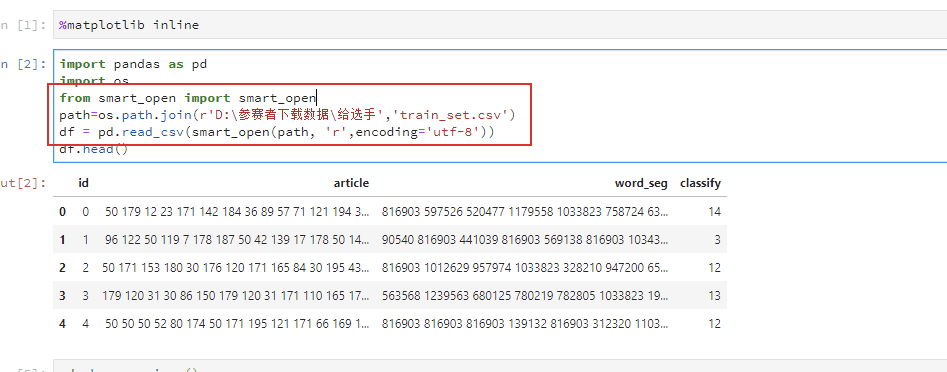python读取大数据 相关内容

2019-09-10 18:20:18 zhangcongyi420 阅读数 231
• ###### python数据分析与机器学习实战【2019新版】

购买课程后，请扫码进入学习群，获取唐宇迪老师答疑 Python数据分析与机器学习实战教程，该课程精心挑选真实的数据集为案例，通过python数据科学库numpy,pandas,matplot结合机器学习库scikit-learn完成一些列的机器学习案例。课程以实战为基础，所有课时都结合代码演示如何使用这些python库来完成一个真实的数据案例。算法与项目相结合，选择经典kaggle项目，从数据预处理开始一步步代码实战带大家入门机器学习。学完该课程即可： 1.掌握Python数据科学工具包，包括矩阵数据处理与可视化展示。 2.掌握机器学习算法原理推导，从数学上理解算法是怎么来的以及其中涉及的细节。 3.掌握每一个算法所涉及的参数，详解其中每一步对结果的影响。 4.熟练使用Python进行建模实战，基于真实数据集展开分析，一步步完成整个建模实战任务。

93875 人正在学习 去看看 唐宇迪

## excel生成批量sql语句

1、步骤1 ，准备一条原始的sql语句，比如我这里有一张表叫做temp表，里面的有10个字段，分别是a1 - a10，那么原始的sql语句应该是下面这样的，

``````insert into temp(a1,a2,a3,a4,a5,a6,a7) values ('?','?','?','?','?','?','?');
``````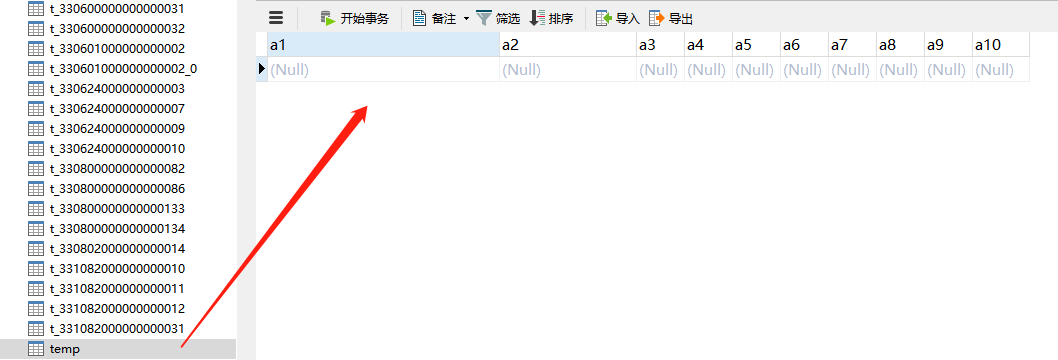2、放在excel中怎么生成insert的sql语句呢？稍微改装一下即可，比如我们要导入到数据库的excel是下面这样的，正好对应了10个字段，由于数据行是从第二行开始的，因此改造成excel的语句如下，

``````=CONCATENATE("insert into temp(a1,a2,a3,a4,a5,a6,a7) values ('"&A2&"','"&B2&"','"&C2&"','"&D2&"','"&E2&"','"&F2&"','"&G2&"');")
``````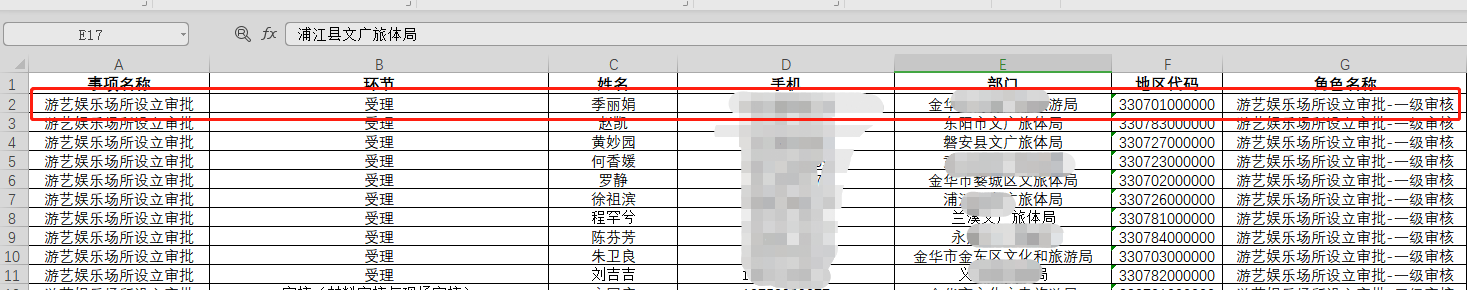3、只需要将这一行语句放到第二行最后一列的下一列即可，然后按回车键，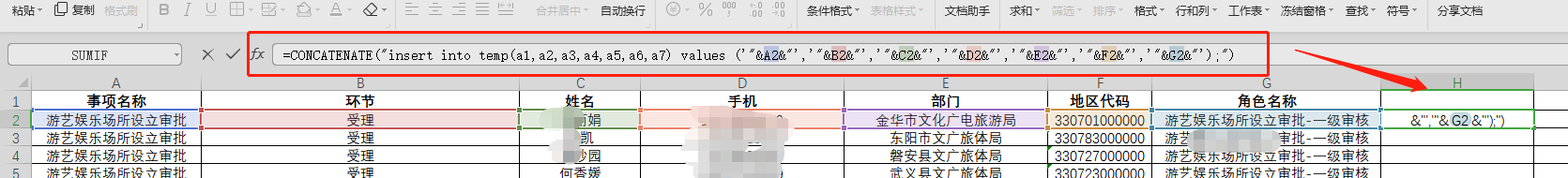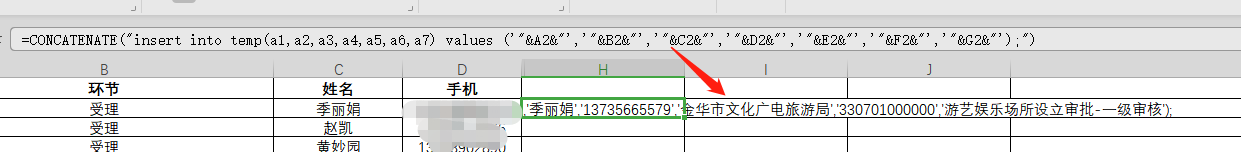``````insert into temp(a1,a2,a3,a4,a5,a6,a7) values ('游艺娱乐场所设立审批','受理','季X娟','137356XX57X','XX广电旅游局','330701000000','游艺娱乐场所设立审批-一级审核');

``````

4、得到了这条sql语句后，基本大功告成了，这时候并不需要每一行执行以下，只需要拖动角落的这个位置一直往下全部选中，此时就可以生成所有的sql语句了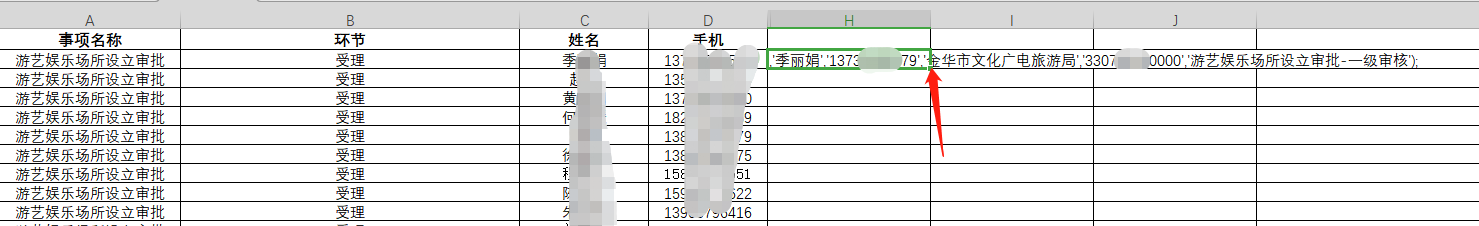## python导入方式

1、单表导入，这里直接贴上代码了

``````
import xlrd
import pymysql

book = xlrd.open_workbook("C:/角色权限文件/金华——人员信息采集表20190910.xlsx")
#sheet = book.sheet_by_index(0);
sheet = book.sheet_by_name("游艺")
#建立mysql的连接
conn = pymysql.connect(
host='你的数据库IP',
user='root',
passwd='数据库密码',
db='数据库名称',
port=3306,
charset='utf8'
)
# 获得游标
cur = conn.cursor()
query = 'insert into temp(a1,a2,a3,a4,a5,a6,a7) values(%s, %s, %s, %s, %s, %s,%s);'
# 创建一个for循环迭代读取xls文件每行数据的, 从第二行开始是要跳过标题行
for r in range(1, sheet.nrows):
a1 = sheet.cell(r,0).value
a2 = sheet.cell(r,1).value
a3 = sheet.cell(r,2).value
a4 = sheet.cell(r,3).value
a5 = sheet.cell(r,4).value
a6 = sheet.cell(r,5).value
a7 = sheet.cell(r,6).value

values = (a1,a2,a3,a4,a5,a6,a7)
# 执行sql语句
cur.execute(query, values)

cur.close()
conn.commit()
conn.close()
columns = str(sheet.ncols)
rows = str(sheet.nrows)
print ("导入 " +columns + " 列 " + rows + " 行数据到MySQL数据库!")

``````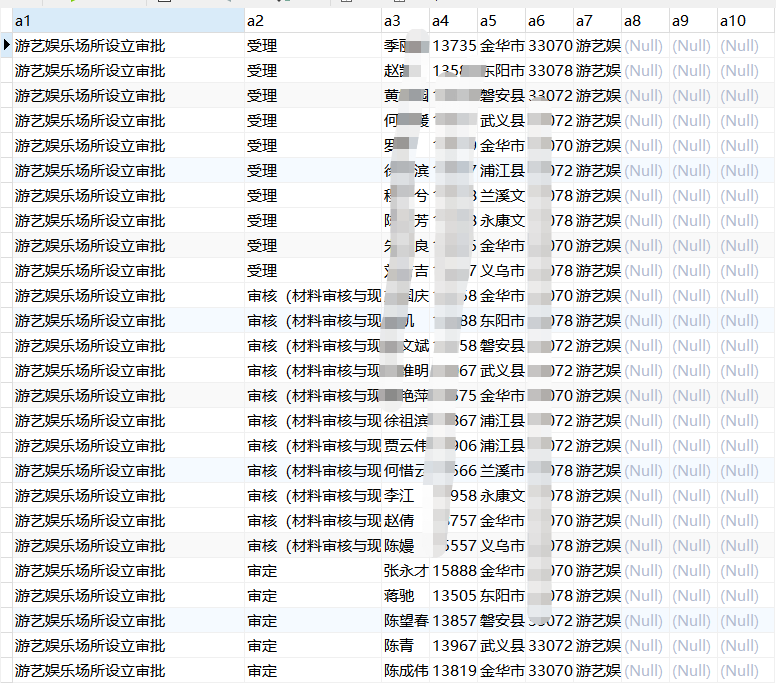``````import xlrd
import pymysql

book = xlrd.open_workbook("C:/角色权限文件/金华——人员信息采集表20190910.xlsx")

#获取所有的esheet
list = book.sheets()
print(list)

sheet_names = book.sheet_names();
print(sheet_names)
print(len(sheet_names))

#建立mysql的连接
conn = pymysql.connect(
host='数据库IP',
user='root',
passwd='密码',
db='库名称',
port=3306,
charset='utf8'
)

# 获得游标
cur = conn.cursor()
query = 'insert into temp(a1,a2,a3,a4,a5,a6,a7) values(%s, %s, %s, %s, %s, %s,%s);'

for i in sheet_names:
sheet_i = book.sheet_by_name(i)

# 创建一个for循环迭代读取xls文件每行数据的, 从第二行开始是要跳过标题行
for r in range(1, sheet_i.nrows):
a1 = sheet_i.cell(r, 0).value
a2 = sheet_i.cell(r, 1).value
a3 = sheet_i.cell(r, 2).value
a4 = sheet_i.cell(r, 3).value
a5 = sheet_i.cell(r, 4).value
a6 = sheet_i.cell(r, 5).value
a7 = sheet_i.cell(r, 6).value
values = (a1, a2, a3, a4, a5, a6, a7)
# 执行sql语句
cur.execute(query, values)

cur.close()
conn.commit()
conn.close()

print ("导入成功")
``````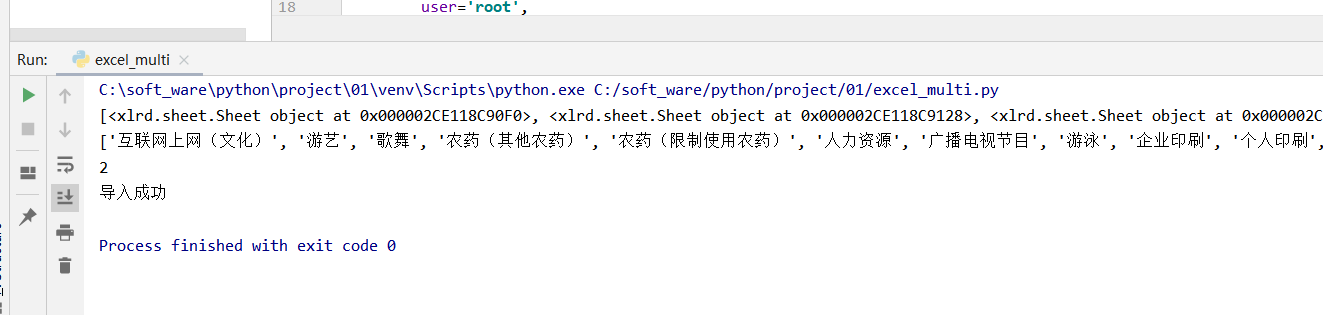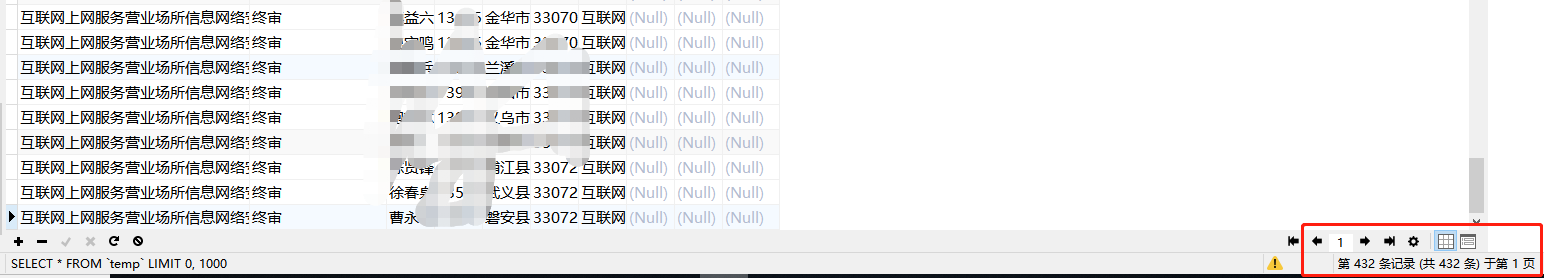python读取大数据 相关内容

2017-01-08 18:09:15 Yan456jie 阅读数 1782
• ###### python数据分析与机器学习实战【2019新版】

购买课程后，请扫码进入学习群，获取唐宇迪老师答疑 Python数据分析与机器学习实战教程，该课程精心挑选真实的数据集为案例，通过python数据科学库numpy,pandas,matplot结合机器学习库scikit-learn完成一些列的机器学习案例。课程以实战为基础，所有课时都结合代码演示如何使用这些python库来完成一个真实的数据案例。算法与项目相结合，选择经典kaggle项目，从数据预处理开始一步步代码实战带大家入门机器学习。学完该课程即可： 1.掌握Python数据科学工具包，包括矩阵数据处理与可视化展示。 2.掌握机器学习算法原理推导，从数学上理解算法是怎么来的以及其中涉及的细节。 3.掌握每一个算法所涉及的参数，详解其中每一步对结果的影响。 4.熟练使用Python进行建模实战，基于真实数据集展开分析，一步步完成整个建模实战任务。

93875 人正在学习 去看看 唐宇迪

python2.x和3.x都可以使用pymysql（3.x不支持MySQLdb

```# -*- coding: utf-8 -*-
import pymysql
try:
#获取一个数据库连接，注意如果是UTF-8类型的，需要制定数据库
conn=pymysql.connect(host='localhost',user='root',passwd='root',db='db_weibo',port=3306,charset='utf8')
cur=conn.cursor()#获取一个游标
cur.execute('select * from t_mweibo limit 10')
data=cur.fetchall()
for d in data :
print data

cur.close()#关闭游标
conn.close()#释放数据库资源
except  Exception :print("发生异常")```

python读取大数据 相关内容

2019-09-06 12:50:53 a72944392 阅读数 27
• ###### python数据分析与机器学习实战【2019新版】

购买课程后，请扫码进入学习群，获取唐宇迪老师答疑 Python数据分析与机器学习实战教程，该课程精心挑选真实的数据集为案例，通过python数据科学库numpy,pandas,matplot结合机器学习库scikit-learn完成一些列的机器学习案例。课程以实战为基础，所有课时都结合代码演示如何使用这些python库来完成一个真实的数据案例。算法与项目相结合，选择经典kaggle项目，从数据预处理开始一步步代码实战带大家入门机器学习。学完该课程即可： 1.掌握Python数据科学工具包，包括矩阵数据处理与可视化展示。 2.掌握机器学习算法原理推导，从数学上理解算法是怎么来的以及其中涉及的细节。 3.掌握每一个算法所涉及的参数，详解其中每一步对结果的影响。 4.熟练使用Python进行建模实战，基于真实数据集展开分析，一步步完成整个建模实战任务。

93875 人正在学习 去看看 唐宇迪

# 使用get方式读取数据

``````f=urllib.request.urlopen('http://192.168.11.78/cgi-bin/xxx.cgi?type=0&stop=0&channel=0')
``````

python读取大数据 相关内容